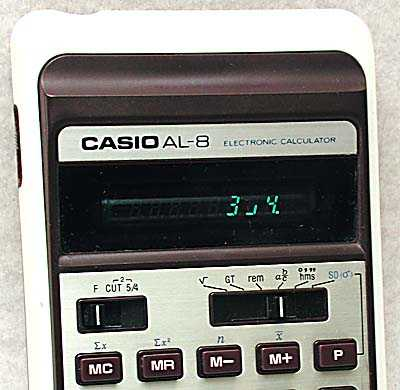# Enumerations¶

## BasicType¶

Basic data type.

Generalisation of the type of data used in data exchange systems, SQL databases, etc. The assumption is to use as few variants as possible, so there is no single character type. Instead, you should use a text type with a character limit. If necessary, a single character can be treated as an integer.

An integer is both an integer and a negative number, as well as a real number. Integers are of course also general numbers.

Date and time are represented by 3 values depending on whether it is date (YYYY-MM-DD), time (hh:mm:ss) or full time stamp (YYYY-MM-DD hh:mm:ss).

```enum Energy.Enumeration.BasicType
{
/// <summary>
/// Text value
/// </summary>
Text,

/// <summary>
/// Any value which is number
/// </summary>
Number,

/// <summary>
/// Integer numbers only
/// </summary>
Integer,

/// <summary>
/// True / False
/// </summary>
Bool,

/// <summary>
/// Date
/// </summary>
Date,

/// <summary>
/// Time
/// </summary>
Time,

/// <summary>
/// Date and time
/// </summary>
Stamp,
}
```

## BooleanStyle¶

Style of representing boolean values.

Used by Energy.Cast.BoolToString().

```enum Energy.Enumeration.BooleanStyle
{
/// <summary>
/// 0/1
/// </summary>
B,

/// <summary>
/// X for true
/// </summary>
X,

/// <summary>
/// V for true
/// </summary>
V,

/// <summary>
/// Y/N
/// </summary>
Y,

/// <summary>
/// T/F
/// </summary>
T,

/// <summary>
/// Yes/No
/// </summary>
/// <remarks>Localised</remarks>
YesNo,

/// <summary>
/// True/False
/// </summary>
/// <remarks>Localised</remarks>
TrueFalse,

/// <summary>
/// 0/1
/// </summary>
/// <remarks>Localised</remarks>
Bit = B,

/// <summary>
/// Y/N
/// </summary>
/// <remarks>Localised</remarks>
YN = Y,

/// <summary>
/// T/F
/// </summary>
/// <remarks>Localised</remarks>
TF = T,
}
```

## MultipleBehaviour¶

Selection of duplicates behaviour.

Specifies behaviour for selecting one element from multiple duplicates.

The default behavior is to overwrite the value and thus taking the last value.

```enum Energy.Enumeration.MultipleBehaviour
{
/// <summary>
/// Unspecified behaviour.
/// </summary>
None,

/// <summary>
/// Take last from duplicates.
/// Setting value will overwrite element if exists.
/// </summary>
Last,

/// <summary>
/// Take first from duplicates.
/// Value may be set only once. It will be ignored if element exists.
/// </summary>
First,
}
```

## RoundingMethod¶

Method of rounding numbers.

```enum Energy.Enumeration.RoundingMethod
{
None = Floor,

/// <summary>
/// Standard method of rounding (HalfUp)
/// </summary>
Standard = HalfUp,

/// <summary>
/// Round down
/// </summary>
Floor = 0,

/// <summary>
/// Round up
/// </summary>
Ceil = 0,

/// <summary>
/// Half Round Up
/// </summary>
HalfUp = 4,

/// <summary>
/// Half Round Down
/// </summary>
HalfDown = 5,

/// <summary>
/// Round to Even (Banker's Rounding)
/// </summary>
ToEven = 2,

/// <summary>
/// Round to Odd.
/// </summary>
ToOdd = 3,
}
```

### Half Round Up¶

This method is commonly used.

However, some programming languages (such as Java, Python) define their half up as round half away from zero here.

http://en.wikipedia.org/wiki/Rounding

```Energy.Base.Enumeration.HalfUp
Energy.Base.Enumeration.Standard
```

### Half Round Down¶

Example: 7.6 rounds up to 8, 7.5 rounds down to 7, 7.4 rounds down to 7.

Anyone remembers the “5/4” mode in vintage calculators?```Energy.Base.Enumeration.HalfDown
```

### Round to Even (Banker’s Rounding)¶

Example: 7.5 rounds up to 8 (because 8 is an even number) but 6.5 rounds down to 6 (because 6 is an even number).

```Energy.Base.Enumeration.ToEven
```

### Round to Odd¶

Example: 7.5 rounds down to 7 (because 7 is an odd number) but 6.5 rounds up to 7 (because 7 is an odd number).

```Energy.Base.Enumeration.ToOdd
```